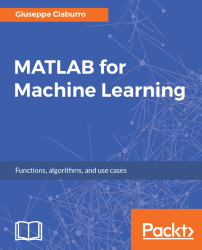•#### MATLAB for Machine Learning#### Overview of this book

MATLAB is the language of choice for many researchers and mathematics experts for machine learning. This book will help you build a foundation in machine learning using MATLAB for beginners. You’ll start by getting your system ready with t he MATLAB environment for machine learning and you’ll see how to easily interact with the Matlab workspace. We’ll then move on to data cleansing, mining and analyzing various data types in machine learning and you’ll see how to display data values on a plot. Next, you’ll get to know about the different types of regression techniques and how to apply them to your data using the MATLAB functions. You’ll understand the basic concepts of neural networks and perform data fitting, pattern recognition, and clustering analysis. Finally, you’ll explore feature selection and extraction techniques for dimensionality reduction for performance improvement. At the end of the book, you will learn to put it all together into real-world cases covering major machine learning algorithms and be comfortable in performing machine learning with MATLAB.
Title PageCreditsForewordwww.PacktPub.comCustomer FeedbackPrefaceFree Chapter
Getting Started with MATLAB Machine LearningImporting and Organizing Data in MATLABFrom Data to Knowledge DiscoveryFinding Relationships between Variables - Regression TechniquesPattern Recognition through Classification AlgorithmsIdentifying Groups of Data Using Clustering MethodsSimulation of Human Thinking - Artificial Neural NetworksImproving the Performance of the Machine Learning Model - Dimensionality ReductionMachine Learning in Practice## Exploratory visualization

In the previous section, we explored the data using numerical indicators; as a result, we learned several methods of finding important information in the distributions under analysis. Now, we will explore another way to extract knowledge from data through a visual approach. By tracing appropriate charts, from our data, we can identify specific trends even before applying machine learning algorithms. What we will be able to draw from this analysis can serve to focus our study on specific areas rather than performing generic simulations.

Through MATLAB tools, we can explore single-variable distributions using univariate plots such as box plots and histograms, for instance. As well, we can show the relationships between variables using bivariate plots such as grouped scatter plots and bivariate histograms. After plotting, we can customize our plot by adding case names, least squares lines, and reference curves.

### The Data Statistics dialog box

We will begin our visual analysis...